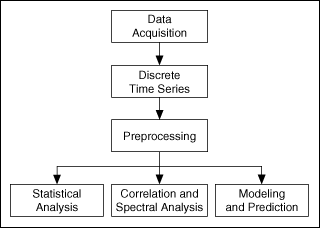# Overview of LabVIEW Time Series Analysis Tools (Advanced Signal Processing Toolkit)

LabVIEW 2014 Advanced Signal Processing Toolkit Help

Edition Date: June 2014

Part Number: 372656C-01

»View Product InfoDownload Help (Windows Only)

The LabVIEW Time Series Analysis Tools provide a collection of VIs that assists you in analyzing scientific and engineering time series and rapidly deploying engineering applications based on the analysis results. You can use these VIs to handle discrete univariate and multivariate (vector) time series.

## Time Series Analysis Procedure

The following diagram illustrates the procedure that you can follow when using the Time Series Analysis Tools to analyze a time series.A typical time series analysis procedure includes the following steps:

1. Acquire a discrete time series through NI-DAQ or by loading existing data from a file.
2. Preprocess the time series if necessary; for example, you can resample the time series using a different time interval, or remove a low-frequency trend from the time series.
3. Obtain useful information from the preprocessed time series by selecting suitable time series analysis methods.

## Time Series Analysis Methods

The Time Series Analysis Tools categorize time series analysis into the following methods:

• Statistical analysis methods—Estimate the statistical parameters of a univariate or multivariate (vector) time series, such as the mean, variance, and skewness values. You can use the estimated statistical parameters to investigate the stochastic characteristics of a physical system that generates time series. The Time Series Analysis Tools also provide methods for analyzing multivariate time series, such as covariance matrix, independent component analysis (ICA), and principal component analysis (PCA).
• Modeling and prediction methodsBuild dynamic models for a univariate or multivariate (vector) time series and perform predictions based on the estimated models. Building a model helps you understand the characteristics of a physical system that generates time series. Performing a prediction helps you monitor and control the movements of a physical system.
• Correlation and spectral analysis methods—Detect hidden periodicity or frequency characteristics in a time series or explain the relationship between two time series. The spectral analysis methods help you identify the frequency components in a time series and extract the characteristics of the physical system that generates the time series. You also can estimate the bispectrum, which is useful for detecting nonlinearities in a time series, and the cepstrum, which is useful for deconvolving a time series.

## Choosing an Appropriate Method

Each of the time series analysis methods is classified as either time domain or frequency domain. You can select appropriate methods from these two classes according to the analysis objective.

All of the statistical analysis methods, the modeling and prediction methods, and the correlation methods discussing in this help are time-domain methods. You can use the statistical analysis methods to investigate the stochastic characteristics of a time series. Stochastic characteristics, for example, are helpful in quality controls in manufacturing production. If you have two or more related time series, you can analyze them jointly using a covariance matrix, PCA, or cross-correlation method to investigate their relatedness. ICA can separate independent signals from linearly mixed data. The modeling methods help you build parametric behavioral models for time series, which help you predict or control future values.

The spectral analysis methods are frequency-domain methods. You can use the nonparametric or model-based spectral analysis methods to investigate the vibration characteristics of physical systems, such as resonance frequencies and harmonic frequencies. Some of the methods also support multivariate time series, such as the MUSIC method, which computes the common spectral components existing in a multivariate vibration time series.

Before applying an analysis method to a time series, you need to preprocess the signal. For example, you need to make sure that the signal contains no low-frequency trends, that the frequency bandwidth is sufficiently narrow, and that the sampling rate is sufficiently high. Use the Preprocessing VIs to preprocess a time series.

The Time Series Analysis Tools also provide a group of Utilities VIs that you can use to generate time series samples, to scale to an engineering unit, to average the power spectrum, or to load pre-stored data from a file.